﻿ Charting Trendlines# Charting Trendlines

Trendlines are an important tool used for analyzing data. They alert you with the general rate of increase or decrease of your Y data over your X data. A common scenario is measuring the rate change of sales price over time. Trendlines can be used to forecast data and therefore lend themselves perfectly to data analysis.

Trendlines are typically used in line, bar or scatter charts. And they are typically auto-generated from your charting software, such as Microsoft Excel. The ComponentOne Chart controls include built-in trend line automation for ease of use. There are several types of trendlines supported, divided into two groups: regression and non-regression. Non-regression trend lines include average, minimum, maximum and moving average (the average over a specified time). Regression trend lines include polynomial, exponential, logarithmic, power and Fourier functions that approximate the data which the functions trend.

In this blog post we will look at the different types of trend lines and you will learn how to add them to C1Chart for WPF (and note that the same techniques work in C1Chart for Silverlight too).

## Types of Trendlines

Linear

A linear trendline is a best-fit straight light. Your data is linear if the data point pattern resembles a line, and a linear trendline is a good fit if the R-squared value is at or near 1.

Polynomial

Polynomial trendlines are curved lines that are used with fluctuating data. They are useful for analyzing gains or losses over a large data set. When using a polynomial trendline, it is important to also set the Order of the line, which can be determined by the number of fluctuations (hills and valleys) in the data. An Order 2 polynomial trendline has only one hill or valley, an Order 3 polynomial trendline has two hills or valleys and so on.

Moving Average

A moving average trendline smoothes out fluctuations in data to show a pattern or trend more clearly.

Other types: Power, Exponential, Logarithmic, Fourier. See here for more details.

## Working with Trendlines in C1Chart

The following chart application was created using C1Chart for WPF, and it shows the average price of gasoline in the United States over the past 20 years. The data is loaded from a modified Excel spreadsheet found here: http://www.eia.doe.gov/petroleum/data_publications/wrgp/mogas_history.html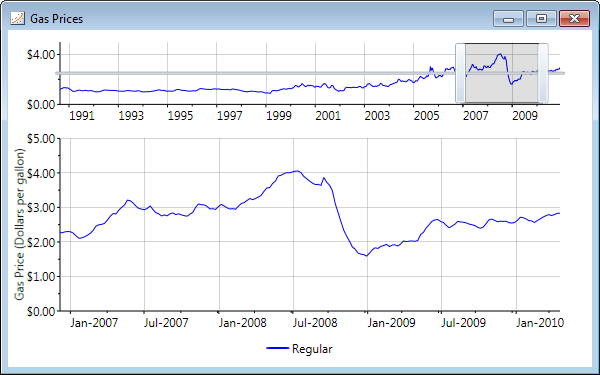(Download Sample) We will use this sample as a starting point for working with trendlines.

You work with trendlines in C1Chart just as you do data series. You can add any multitude of data series to a C1Chart by instantiating a series and adding it to the chart's data collection. Trendlines work the same way and come with some special settings. You bind trendlines to your data collection in the same way you do a data series. The following code creates a data series and a default trendline.

Note: The TrendLine class is included in the C1.WPF.C1Chart.Extended library so you need to add this reference to your project before you begin working with them.

``````//add data series
XYDataSeries ds = new XYDataSeries();
ds.Label = "Series";
ds.XValuesSource = myXValues;
ds.ValuesSource = myValues;

TrendLine tl = new TrendLine();
tl.Label = "Trendline";
tl.ConnectionStroke = new SolidColorBrush(Colors.Red);
tl.XValuesSource = myXValues;
tl.ValuesSource = myValues;

``````

You can see by the code above that adding a trendline is very similar to adding a second data series. C1Chart will automatically calculate and plot the trendline based upon the values. By default, a trendline has an Order 2 polynomial fit type.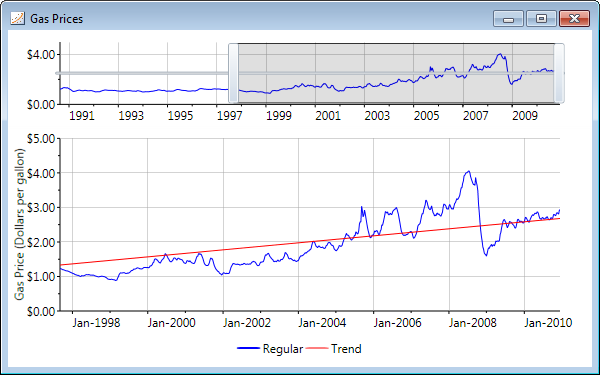We can change other properties of the TrendLine such as FitType and Order. For example, here we add a polynomial trendline with Order 6 (this is a closer fit but still not close enough for this data set).

``````TrendLine tl = new TrendLine();
tl.Label = "Trend";
tl.FitType = FitType.Polynom;
tl.Order = 6;
...
``````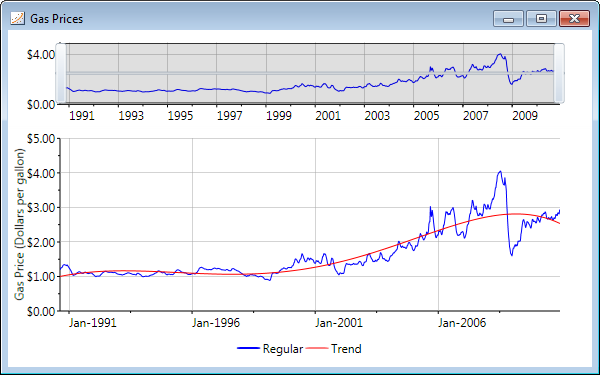### Non-regression Trendlines

Use non-regression trendlines, such as average, minimum and maximum, to mark certain simple characteristics in your data. For example, you can show an average trendline which draws a straight, horizontal or vertical line on your chart at wherever the average X or Y value is. To do this we just set the FitType property of each trendline to AverageX, AverageY, MaxX, MaxY, MinX or MinY.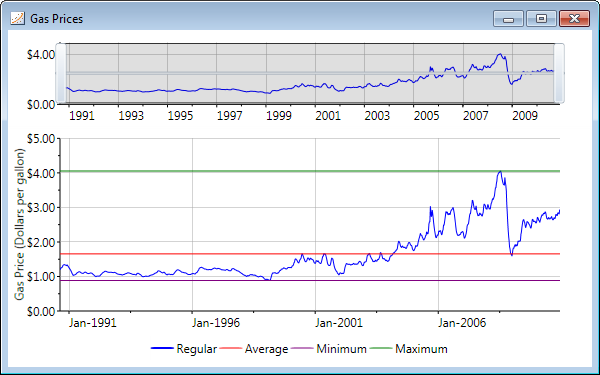### Moving Average

Given the gas price data set above, a moving average trendline might be the best option. A moving average trendline uses a specific number of data points (set by the Period property), averages them, and uses the average value as a point in the trendline. For example, if Period is set to 2, then the average of the first two data points is used as the first point in the moving average trendline. The average of the second and third data points is used as the second point in the trendline, and so on. So if our data set has a total of 100 points, and we set the Period to 10, our moving average trendline will calculate the average value for each 10 points and plot.

To set up a moving average trendline in C1Chart we instantiate a MovingAverage object, set the Period property and set remaining properties as we do for trendlines and data series.

``````MovingAverage ma = new MovingAverage();
ma.Label = "Moving Average";
ma.Period = 48;
ma.XValuesSource = days;
ma.ValuesSource = price;
ma.ConnectionStroke = new SolidColorBrush(Colors.Red);
``````

The gas price data set has values for every 7 days, so a Period value of 48 would mean each year of data is averaged. This will give a much closer fitting trendline.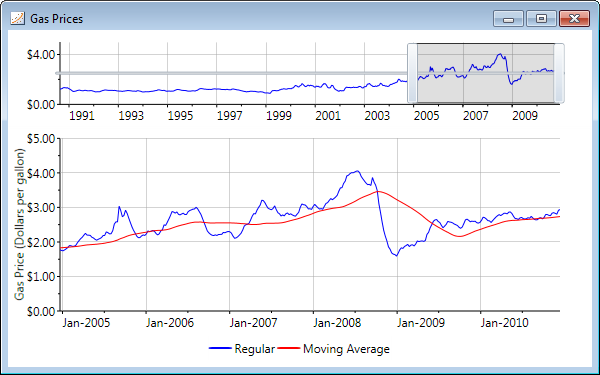## Conclusion

Trendlines are a lot more fun when you don't have to really do much work to generate them on your charts. C1Chart includes about 10 different types of trendlines built in; all you have to do is control how they are added to the charts and what they look like!Greg Lutz